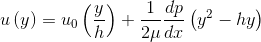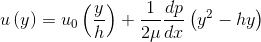# Problem with units

## Homework Statement

The velocity profile between two plates separated by a distance, h=2 {m}, has a flow distribution given by:The dynamic viscosity of the fluid is given as 1.5E-1 {Pa s}. The upper wall is moving at velocity u0 of 7 {m/s}. The pressure gradient dp/dx is -0.015 {Pa/m}. Find the shear stress at y=0.8 {m}.

Input your answer to in {N/m2} or {Pa} to four decimal places.

## The Attempt at a Solution

I feel like this problem should be just plug and chug, but my units aren't working out to be in Pa.
u(0.8m)=(7m/s)(0.8m/2m)+(1/(2*(1.5E-1)Pa*s))(-0.015Pa/m)((0.8m)2-1.6m2)
I keep getting 2.85 m/s

SteamKing
Staff Emeritus
Homework Helper

## Homework Statement

The velocity profile between two plates separated by a distance, h=2 {m}, has a flow distribution given by:The dynamic viscosity of the fluid is given as 1.5E-1 {Pa s}. The upper wall is moving at velocity u0 of 7 {m/s}. The pressure gradient dp/dx is -0.015 {Pa/m}. Find the shear stress at y=0.8 {m}.

Input your answer to in {N/m2} or {Pa} to four decimal places.

## The Attempt at a Solution

I feel like this problem should be just plug and chug, but my units aren't working out to be in Pa.
u(0.8m)=(7m/s)(0.8m/2m)+(1/(2*(1.5E-1)Pa*s))(-0.015Pa/m)((0.8m)2-1.6m2)
I keep getting 2.85 m/s
Remember u(y) is the velocity profile of the fluid, not the shear stress, so units of m/s are consistent.

What is the formula for shear stress in a fluid?

https://en.wikipedia.org/wiki/Shear_stress

•jdawg
Ohh ok! I guess I should've read the question a little more closely. Did you end up with 0.5344 Pa?

SteamKing
Staff Emeritus
Homework Helper
Ohh ok! I guess I should've read the question a little more closely. Did you end up with 0.5344 Pa?
I didn't solve the problem. I merely noted that you had confused the formula for the velocity profile with the formula for shear stress.

Oh ok, do you think that the value I got is reasonable?

SteamKing
Staff Emeritus
Homework Helper
Oh ok, do you think that the value I got is reasonable?
I have no idea without solving the problem myself.

Since you have apparently done the work, why don't you post it?

τ(y)=μ(u/y)
τ(y)=(1.5E-1 Pa*s)((2.85 m/s)/(0.8m))=0.5344 Pa

SteamKing
Staff Emeritus
•